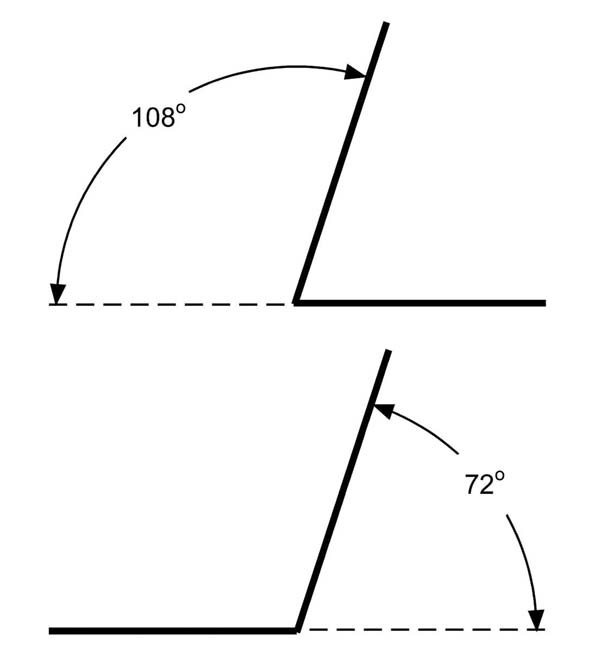The Pentagonal SystemPent Angles make pentagons, or a 72o transition.
Pentagonal complements are a 108o transition.

• 12 sizes: 1" to 12" in 1 inch increments.
• Labeled: size is stamped into the metal for easy identification.
• Oversized: sized up 1/8" to include a layer of fiber.
• Stainless steel: long life, stable in the kiln.
• Light guage material: easily make other shapes or true up old ones.

Pent (72o) Equal Leg Angles

 SC_A72_1 #1 equal leg angle \$ 2.00
 SC_A72_2 #2 equal leg angle \$ 3.20
 SC_A72_3 #3 equal leg angle \$ 4.40
 SC_A72_4 #4 equal leg angle \$ 5.60
 SC_A72_5 #5 equal leg angle \$ 6.80
 SC_A72_6 #6 equal leg angle \$ 8.00
 SC_A72_7 #7 equal leg angle \$ 9.20
 SC_A72_8 #8 equal leg angle \$ 10.40
 SC_A72_9 #9 equal leg angle \$ 11.60
 SC_A72_10 #10 equal leg angle \$ 12.80
 SC_A72_11 #11 equal leg angle \$ 14.00
 SC_A72_12 #12 equal leg angle \$ 15.20

Pent Complements (108o) Equal Leg Angles

 SC_A108_1 #1 equal leg angle \$ 2.00
 SC_A108_2 #2 equal leg angle \$ 3.20
 SC_A108_3 #3 equal leg angle \$ 4.40
 SC_A108_4 #4 equal leg angle \$ 5.60
 SC_A108_5 #5 equal leg angle \$ 6.80
 SC_A108_6 #6 equal leg angle \$ 8.00
 SC_A108_7 #7 equal leg angle \$ 9.20
 SC_A108_8 #8 equal leg angle \$ 10.40
 SC_A108_9 #9 equal leg angle \$ 11.60
 SC_A108_10 #10 equal leg angle \$ 12.80
 SC_A108_11 #11 equal leg angle \$ 14.00
 SC_A108_12 #12 equal leg angle \$ 15.20